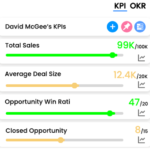## Key Performance Indicators Examples#### Annual Return

Annual return refers to the amount of money that an investment earns over a one-year period, typically expressed as a percentage of the initial investment. It is a measure of the profitability of an investment and can be used to compare the performance of different investments. The annual return can be calculated by taking the total return over a year (the ending value of the investment minus the initial investment) and dividing it by the initial investment, then multiplying by 100 to express it as a percentage.
The formula for calculating annual return is:

(Ending Value – Beginning Value) / Beginning Value x 100 = Annual Return (%)

##### Where:
• Ending Value is the final value of the investment at the end of the period.
• Beginning Value is the initial value of the investment at the start of the period.

For example, if an investment starts at \$1000 and ends at \$1200, the annual return would be:

(\$1200 – \$1000) / \$1000 x 100 = 20%

Alternatively, the formula can be computed by taking the natural logarithm of the final value divided by the initial value, then multiply by the number of compounding periods per year.

ln(Ending Value/Beginning Value) * compounding periods per year = Annual Return(%)

Please note that this formula assumes that the returns are reinvested back into the investment and that the returns are compounded.